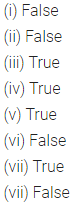# ML Aggarwal Class 6 Solutions for ICSE Maths Chapter 4 Playing with Numbers Ex 4.2

## ML Aggarwal Class 6 Solutions for ICSE Maths Chapter 4 Playing with Numbers Ex 4.2

Question 1.
Which of the following numbers are divisible by 5 or by 10:
(i) 3725
(ii) 48970
(iii) 56823
(iv) 760035
(v) 7893217
(vi) 4500010
Solution:Question 2.
Which of the following numbers are divisible by 2, 4 or 8:
(i) 54014
(ii) 723840
(iii) 6531088
(iv) 75689604
(v) 786235
(vi) 5321048
Solution: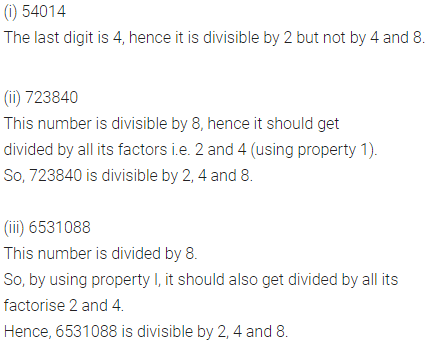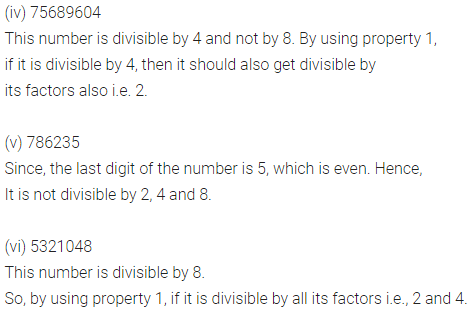Question 3.
Which of the following numbers are divisible by 3 or 9:
(i) 7341
(ii) 59031
(iii) 12345678
(iv) 560319
(v) 720634
(vi) 3721509
Solution: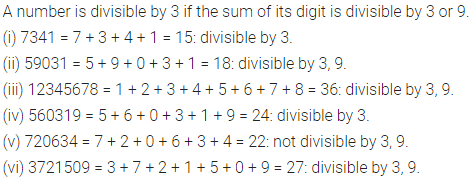Question 4.
Examine the following numbers for divisibility by 11:
(i) 10428
(ii) 70169803
(iii) 7136985
Solution: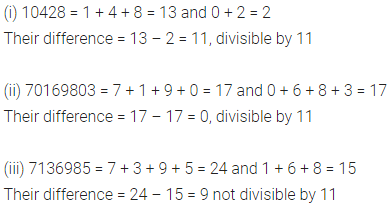Question 5.
Examine the following numbers for divisibility by 6:
(i) 93573
(ii) 217944
(iii) 5034126
(iv) 901352
(v) 639210
(vi) 1790184
Solution: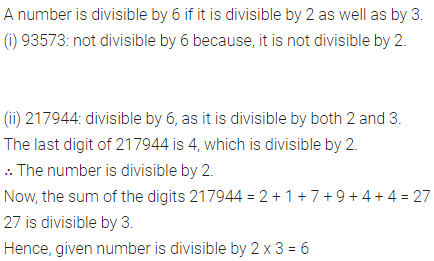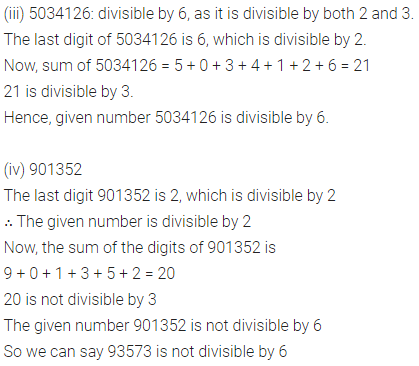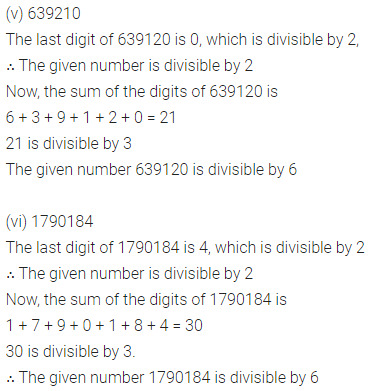Question 6.
In each of the following replace ‘*’ by a digit so that the number formed is divisible by 9:
(i) 4710 * 82
(ii) 70 * 356722
Solution: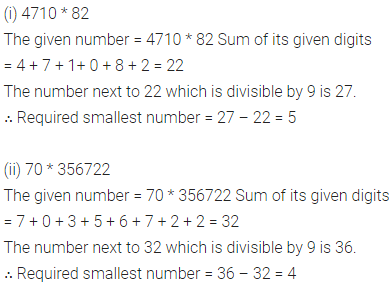Question 7.
In each of the following replace ‘*’ by (i) the smallest digit (ii) the greatest digit so that the number formed is divisible by 3:
(a) 4 * 672
(b) 4756 * 2
Solution:Question 8.
In each of the following replace ‘*’ by a digit so that the number formed is divisible by 11:
(i) 8 * 9484
(ii) 9 * 53762
Solution: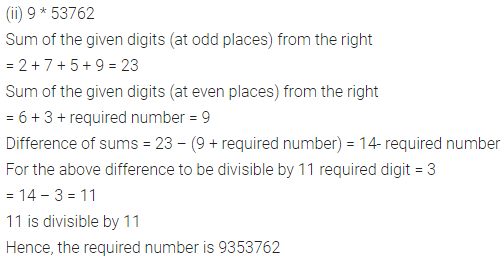Question 9.
In each of the following replace ‘*’ by (i) the smallest digit 00 the greatest digit so that the number formed is divisible by 6:
(a) 2 * 4706
(b) 5825 * 34
Solution: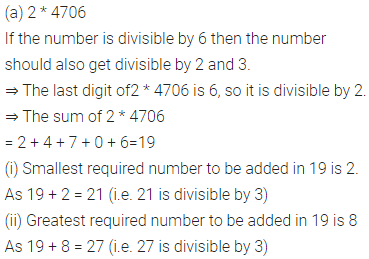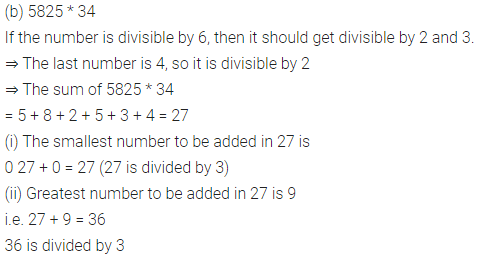Question 10.
Which of the following numbers are prime:
(i) 101
(ii) 251
(iii) 323
(iv) 397
Solution: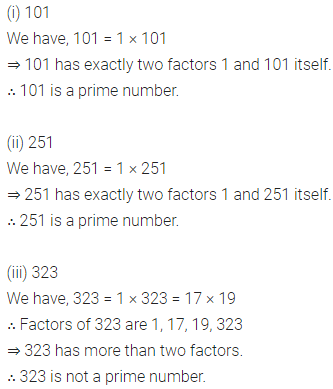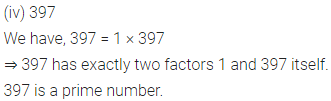Question 11.
Determine if 372645 is divisible by 45.
Solution:Question 12.
A number is divisible by 12. By what other numbers will that number be divisible?
Solution:Question 13.
A number is divisible by both 3 and 8. By which other numbers will that number be always divisible?
Solution:Question 14.
State whether the following statements are true (T) or false (F):
(i) If a number is divisible by 4, it must be divisible by 8.
(ii) If a number is divisible by 3, it must be divisible by 9.
(iii) If a number is divisible by 9, it must be divisible by 3.
(iv) If a number is divisible by 9 and 10 both, it must be divisible by 90.
(v) If a number divides two numbers separately, then it must divide their sum.
(vi) If a number divides the sum of two numbers, then it must divide the two numbers separately. .
(vii) If a number is divisible by 3 and 8 both, it must be divisible by 12.
(viii) If a number is divisible by 6 and 15 both, it must be divisible by 90.
Solution: